# Octagons - polygons with 8 sides

By Martin McBride, 2022-10-10
Tags: octagon 8 sided shape regular polygon irregular polygon
Categories: gcse geometryA octagon is a flat shape with 8 straight sides.

## Regular and irregular octagons

A regular octagon is a 8-sided shape where every side is the same length and every corner has the same angle. All regular octagons have the same shape, like this: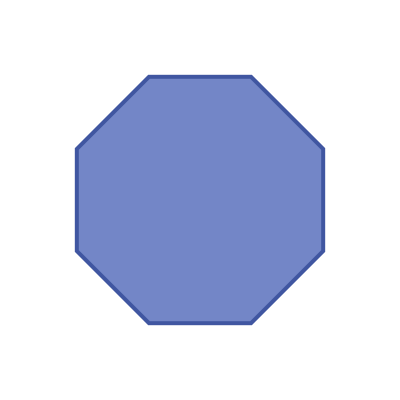An irregular octagon is a 8 sides shape where not every side and angle are equal. There are many different irregular octagon shapes, here is an example: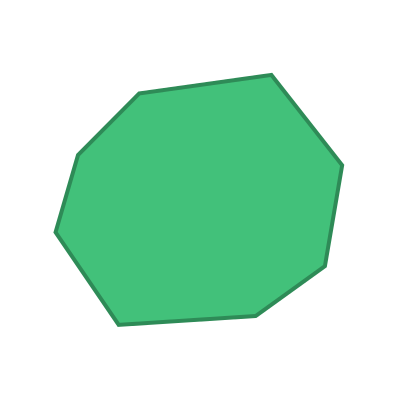## Name

The name octagon is from the Greek octagonon (meaning octagon).

Octagons are sometimes called 8-gons.

## Interior angles

The interior angles of a octagon are shown here: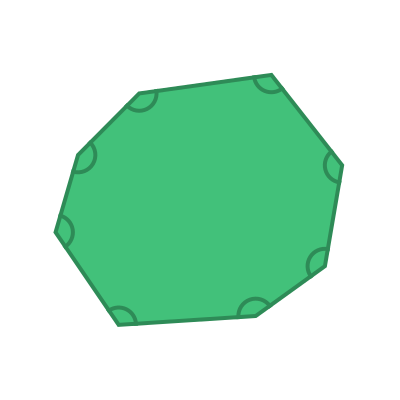The sum of these 8 angles is given by the formula:

sum of interior angles = (n - 2) x 180


Where n is the number of sides. In this case, the number of sides n is 8, so the sum of the interior angles is:

(8 - 2) x 180 = 1080 degrees


For a regular octagon, all the interior angles are equal: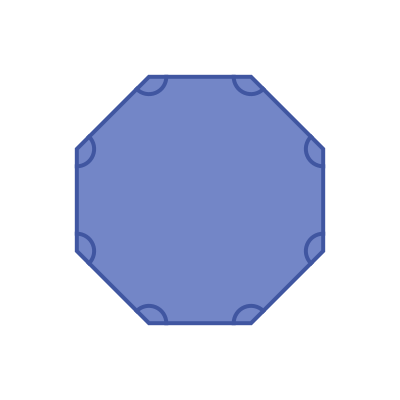This means that the interior angle of a regular octagon is:

1080 / 8 =  135 degrees


## Exterior angles

The exterior angles of a octagon are shown here: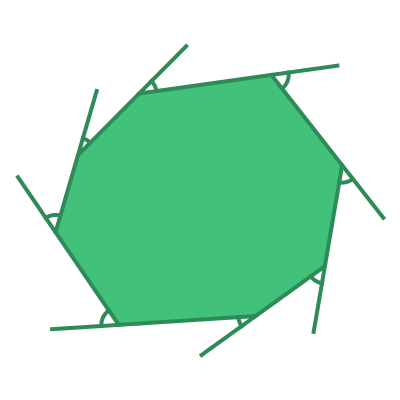The sum of the exterior angles of any polygon is 360 degrees.

For a regular octagon, all the interior angles are equal: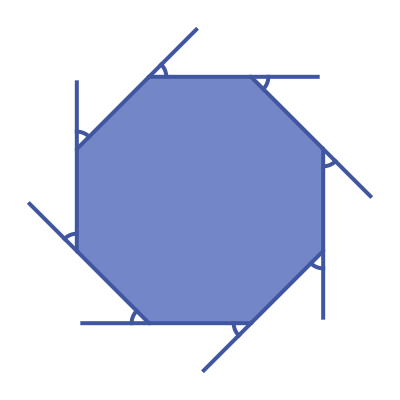This means that the exterior angle of a regular octagon is:

360 / 8 =  45 degrees


## Symmetry of regular octagons

A regular octagon has 8 lines of symmetry. This means that it can be reflected over each of the 8 grey lines shown here: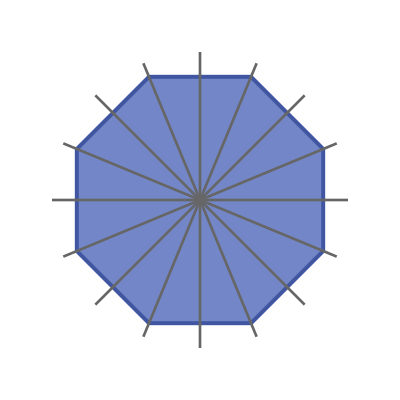A regular octagon has rotational symmetry of order 8. This means that if it rotated about its centre by a 8th of a full turn, it will map onto itself: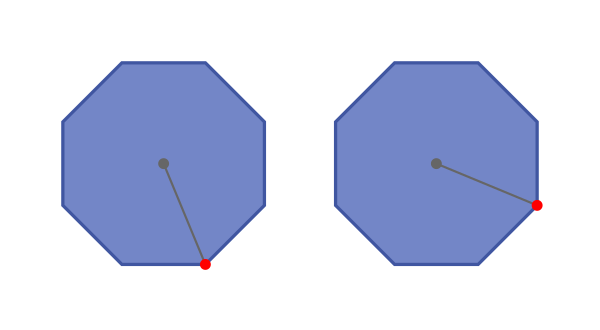## Real life examples

Octagons are common design elements: signs (traffic Stop signs are octagonal in many countries), floor tiles, biscuit tins, even quirky window frames. This is partly because the angles in a regular octagon are all multiples of 45 degrees which often makes items of that shape easier to manufacture.